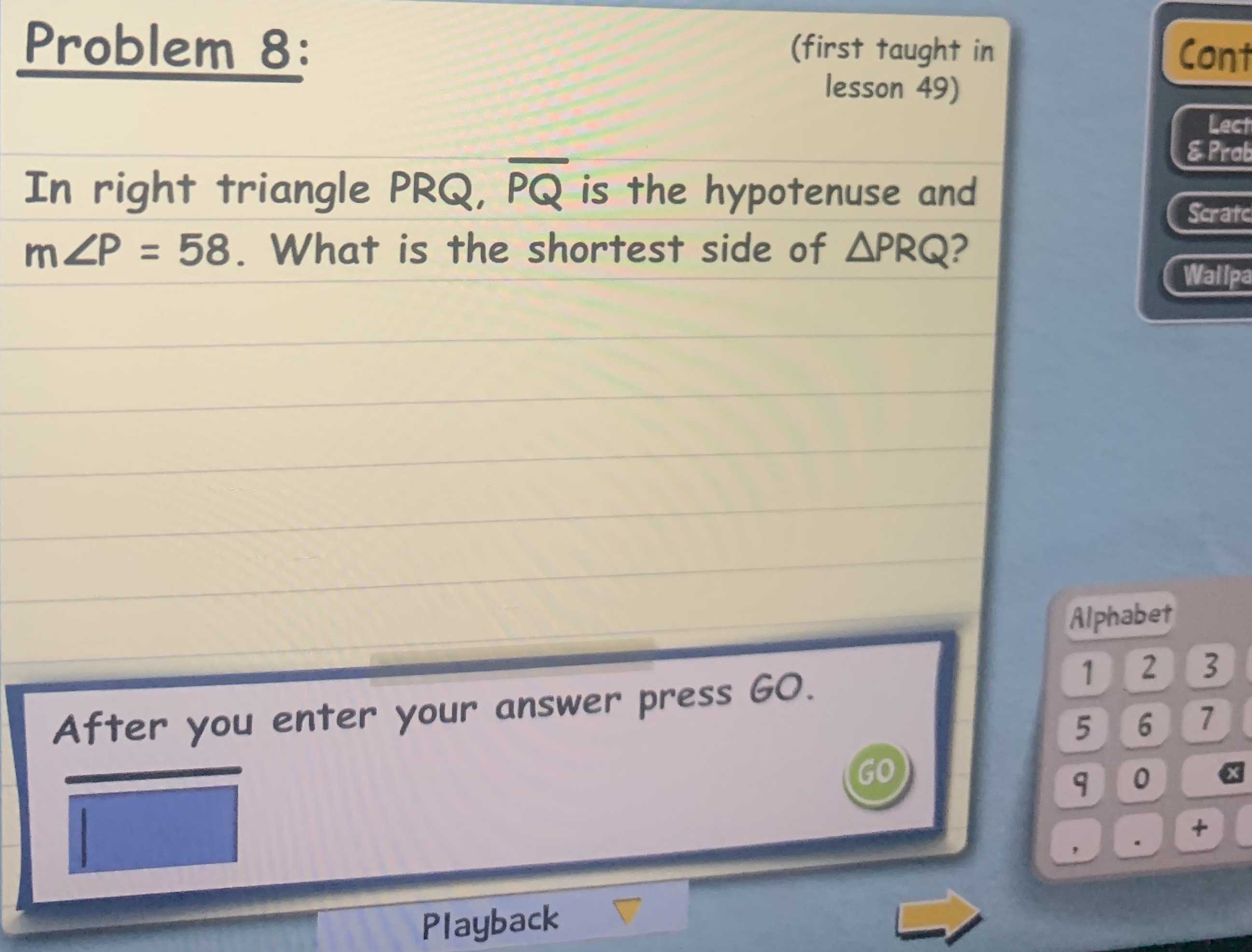### Still have math questions?In right triangle $$P R Q , \overline { P Q }$$ is the hypotenuse and $$m \angle P = 58$$ . What is the shortest side of $$\triangle P R Q ?$$